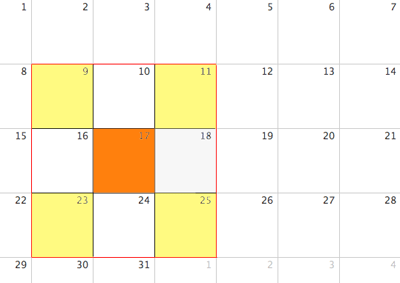Choose two different blocks of nine numbers arranged 3 across and 3 down on a calendar, and check that this rule holds: For any block of nine numbers the average of the four corner numbers is equal to the middle number. Show your work and explain why the rule works.

Thank you!!

Hi,

Did you try it? This is the block of 9 numbers that I tried.The average of the 4 corner numbers is

(9 + 11 + 23 + 25)/4 = 68/4 = 17

and 17 is the middle number.

Why does it work?

Spread the 3 rows of the calendar into one row.

8 9 10 11 12 13 14 15 16 17 18 19 20 21 22 23 24 25 26 27 28

Can you see now why 17 is the average of the 4 corner numbers?

Penny